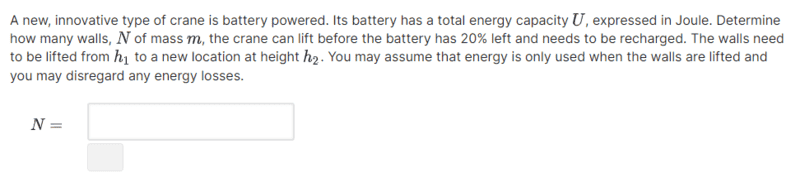# Energy required to lift walls

Thickmax
Homework Statement:
Pre University qualification top up course
Relevant Equations:
See belowwork = mass * gravity * change in height

w = m*g*(h2 - h1)

Total energy = Sum of potential energy + Kinetic Energy

work = 1/2Kinetic Energy * Potential energy ^2

w = (1/2 * K) * U^2

m*g*(h2-h1)=(1/2*k)*U^2

rearanged = (2(m*g*(h2-h1))/U^2)=K

We are given

U, m, g, h2 and h1

Firstly, is my above working along the right line.

Secondly, how to I show this as 80%?

Mentor
work = mass * gravity * change in height

w = m*g*(h2 - h1)
Keep it simple. That's the work done -- and thus the energy expended -- to lift one wall. So, how much energy is needed to lift N walls? Set that equal to 80% of the battery's energy to see how many can be lifted.

Don't worry about KE. Just assume the walls are lifted without any appreciable increase in KE.

Last edited:
•Thickmax
Thickmax
I've never seen that equation before. What are the units of both sides?Imagine that you lift each wall so slowly that it's kinetic energy can always be taken as zero. The work done by the battery to raise a single wall is ##mg(h_2-h_1)##, as you wrote. How much work does the battery then do to raise ##N## walls; and to what fraction of the number ##U## does this correspond?
If you've never seen it before, I've probably not done it right!

W=F*Displacement

Integrated = K*potential energy

Integrated = 1/2*K*potential energy^2

Mentor
W=F*Displacement
That makes sense. It's the definition of work.

But the following does not make sense. What are you integrating?
Integrated = K*potential energy

Integrated = 1/2*K*potential energy^2

Thickmax
Keep it simple. That's the work done -- and thus the energy expended -- to lift one wall. So, how much energy is needed to lift N walls? Set that equal to 80% of the battery's energy to see how many can be lifted.

Don't worry about KE. Just assume the walls are lifted without any appreciable increase in KE.
ok, so N(m*g*(h2 - h1))=U?

and I need to rearrange (N(m*g*(h2-h1))=U

N=U/(m*g*(h2-h1)) right?

Mentor
ok, so N(m*g*(h2 - h1))=U?

and I need to rearrange (N(m*g*(h2-h1))=U

N=U/(m*g*(h2-h1)) right?
Almost. Don't set the energy needed equal to U. U is the total capacity of the battery -- you only want to use a fraction of that total.

Thickmax
Ok, so...

(N(m*g*(h2-h1))=4/5U

N=4/5U/(m*g*(h2-h1))

Mentor
Now you've got it.

Thickmax
Now you've got it.
Mind blown how it makes sense now!

Thank you soo much for your help